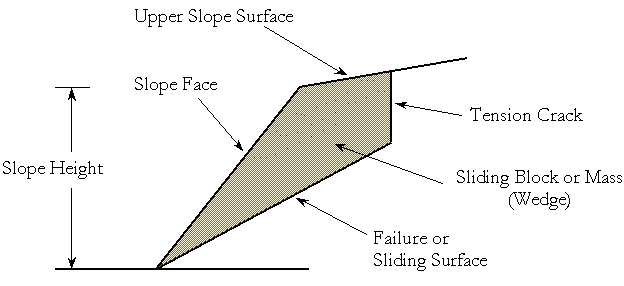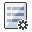Expert Modelling: Hands-on help from Rocscience Engineers. Register Here

# Input Data Overview

RocPlane computes the Factor of Safety for the sliding of a block / wedge formed in a rock slope by:

1. The face of the slope,
2. A discontinuity that strikes parallel to the slope and daylights into the slope face,
3. The upper ground surface, and
4. A tension crack (optional).

A typical problem geometry in RocPlane is illustrated below.At an early stage in the solution process, RocPlane checks if the geometry of a model is valid before proceeding to calculate a Safety Factor or Probability of Failure for a sliding block. For more information on the validation of model geometry, see Geometry Validation in RocPlane.

To enter model parameters, select the Input Data toolbar buttonor Input Data in the Analysis menu. This opens the Input Data dialog. This dialog allows you to define the following model input parameters: